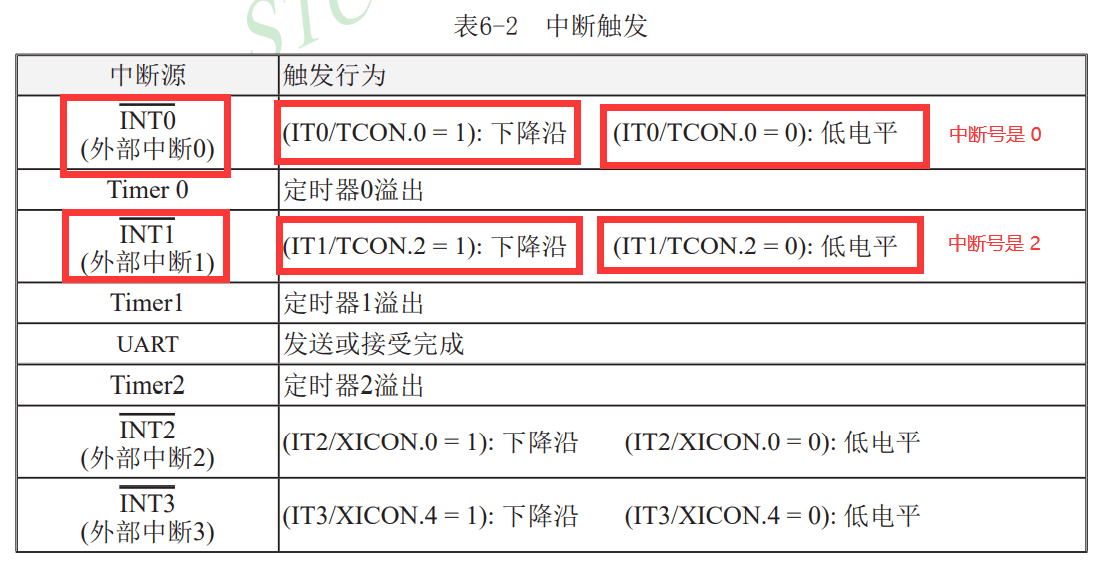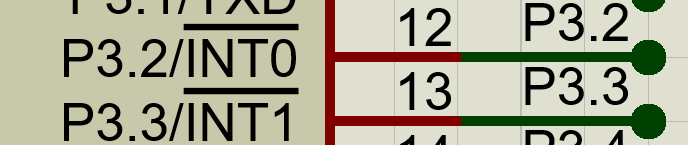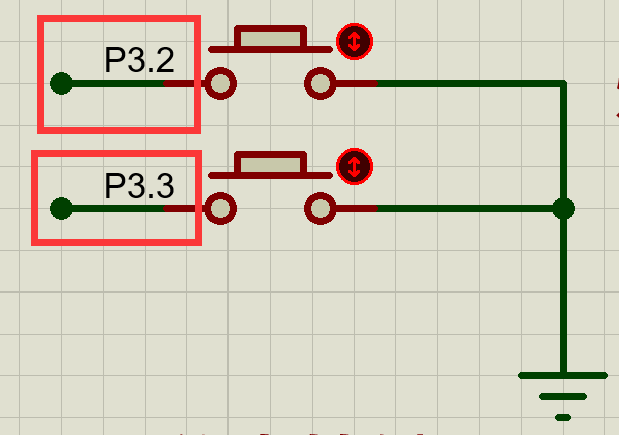# 第三章-51单片机外部中断

## 简述## 1 外部中断初始化

``````void Int0_Init(void)
{
EA = 1; //打开总中断
EX0 = 1; //允许外部中断0触发中断
IT0 = 1; //设置外部中断0触发方式 方式为下降沿触发
}``````

``````void Int1_Init(void)
{
EA = 1;								//打开总中断
EX1 = 1;							//允许外部中断1触发中断
IT1 = 0;							//设置外部中断1触发方式   方式为低电平触发
}``````

``````void main()
{
Int0_Init();												//外部中断0初始化
Int1_Init();												//外部中断1初始化

while(1)
{
Key_function();										//按键函数
Monitor_function();								//监测函数
Display_function();								//显示函数
Manage_function();								//处理函数
}
}``````

## 2 外部中断服务函数，注意中断号是不一样的

``````void Int0_IRQHandler(void) interrupt 0
{
LED = ~LED;
}``````

``````void Int1_IRQHandler(void) interrupt 2
{
LED = ~LED;
Delay_function(500);      //延时0.5秒
}``````

## 3 外部中断的引脚和现象